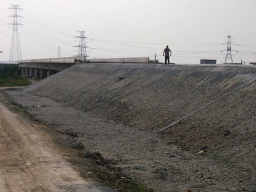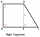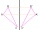# Embankment

The railway embankment 300 m long has a cross section of an isosceles trapezoid with bases of 14 m and 8 m. The trapezoidal arms are 5 m long. Calculate how much m3 of soil is in the embankment?

Correct result:

V =  13200 m3

#### Solution:

$l=300 \ \text{m} \ \\ a=14 \ \text{m} \ \\ c=8 \ \text{m} \ \\ r=5 \ \text{m} \ \\ \ \\ x=\dfrac{ a-c }{ 2 }=\dfrac{ 14-8 }{ 2 }=3 \ \text{m} \ \\ r^2=h^2+x^2 \ \\ h=\sqrt{ r^2-x^2 }=\sqrt{ 5^2-3^2 }=4 \ \text{m} \ \\ S=\dfrac{ a+c }{ 2 } \cdot \ h=\dfrac{ 14+8 }{ 2 } \cdot \ 4=44 \ \text{m}^2 \ \\ V=l \cdot \ S=300 \cdot \ 44=13200 \ \text{m}^3$Our examples were largely sent or created by pupils and students themselves. Therefore, we would be pleased if you could send us any errors you found, spelling mistakes, or rephasing the example. Thank you!

Please write to us with your comment on the math problem or ask something. Thank you for helping each other - students, teachers, parents, and problem authors.Tips to related online calculators
Tip: Our volume units converter will help you with the conversion of volume units.
Pythagorean theorem is the base for the right triangle calculator.

#### You need to know the following knowledge to solve this word math problem:

We encourage you to watch this tutorial video on this math problem:

## Next similar math problems:

• Integer sidesA right triangle with an integer length of two sides has one leg √11 long. How much is its longest side?
• SailboatThe 20 m long sailboat has an 8 m high mast in the middle of the deck. The top of the mast is fixed to the bow and stern with a steel cable. Determine how much cable is needed to secure the mast and what angle the cable will make with the ship's deck.
• Hexagonal pyramidFind the area of a shell of the regular hexagonal pyramid, if you know that its base edge is 5 cm long and the height of this pyramid is 10 cm.
• Triangular prismThe triangular prism has a base in the shape of a right triangle, the legs of which is 9 cm and 40 cm long. The height of the prism is 20 cm. What is its volume cm3? And the surface cm2?
• Hexagonal pyramidFind the volume of a regular hexagonal pyramid, the base edge of which is 12 cm long and the side edge 20 cm.
• The regularThe regular triangular prism has a base in the shape of an isosceles triangle with a base of 86 mm and 6.4 cm arms, the height of the prism is 24 cm. Calculate its volume.
• Calculate 7Calculate the height of the trapezoid ABCD, where coordinates of vertices are: A[2, 1], B[8, 5], C[5, 5] and D[2, 3]A quadrilateral pyramid, which has a rectangular base with dimensions of 24 cm, 13 cm. The height of the pyramid is 18cm. Calculate 1/the area of the base 2/casing area 3/pyramid surface 4/volume of the pyramid
• Hexagonal pyramidCalculate the volume and surface area of a regular hexagonal pyramid with a base edge a = 30 m and a side edge b = 50 m.
• Calculate 8Calculate the coordinates of point B axially symmetrical with point A[-1, -3] along a straight line p : x + y - 2 = 0.
• Truncated cone 6Calculate the volume of the truncated cone whose bases consist of an inscribed circle and a circle circumscribed to the opposite sides of the cube with the edge length a=1.
• Distance of pointsA regular quadrilateral pyramid ABCDV is given, in which edge AB = a = 4 cm and height v = 8 cm. Let S be the center of the CV. Find the distance of points A and S.In a regular quadrilateral pyramid, the height is 6.5 cm and the angle between the base and the side wall is 42°. Calculate the surface area and volume of the body. Round calculations to 1 decimal place.A regular quadrilateral pyramid has a volume of 24 dm3 and a base edge a = 4 dm. Calculate: a/height of the pyramid b/sidewall height c/surface of the pyramidFind the equation of the circle inscribed in the rhombus ABCD where A[1, -2], B[8, -3] and C[9, 4].Calculate the distance of a point A[0, 2] from a line passing through points B[9, 5] and C[1, -1].On a line p : 3 x - 4 y - 3 = 0, determine the point C equidistant from points A[4, 4] and B[7, 1].